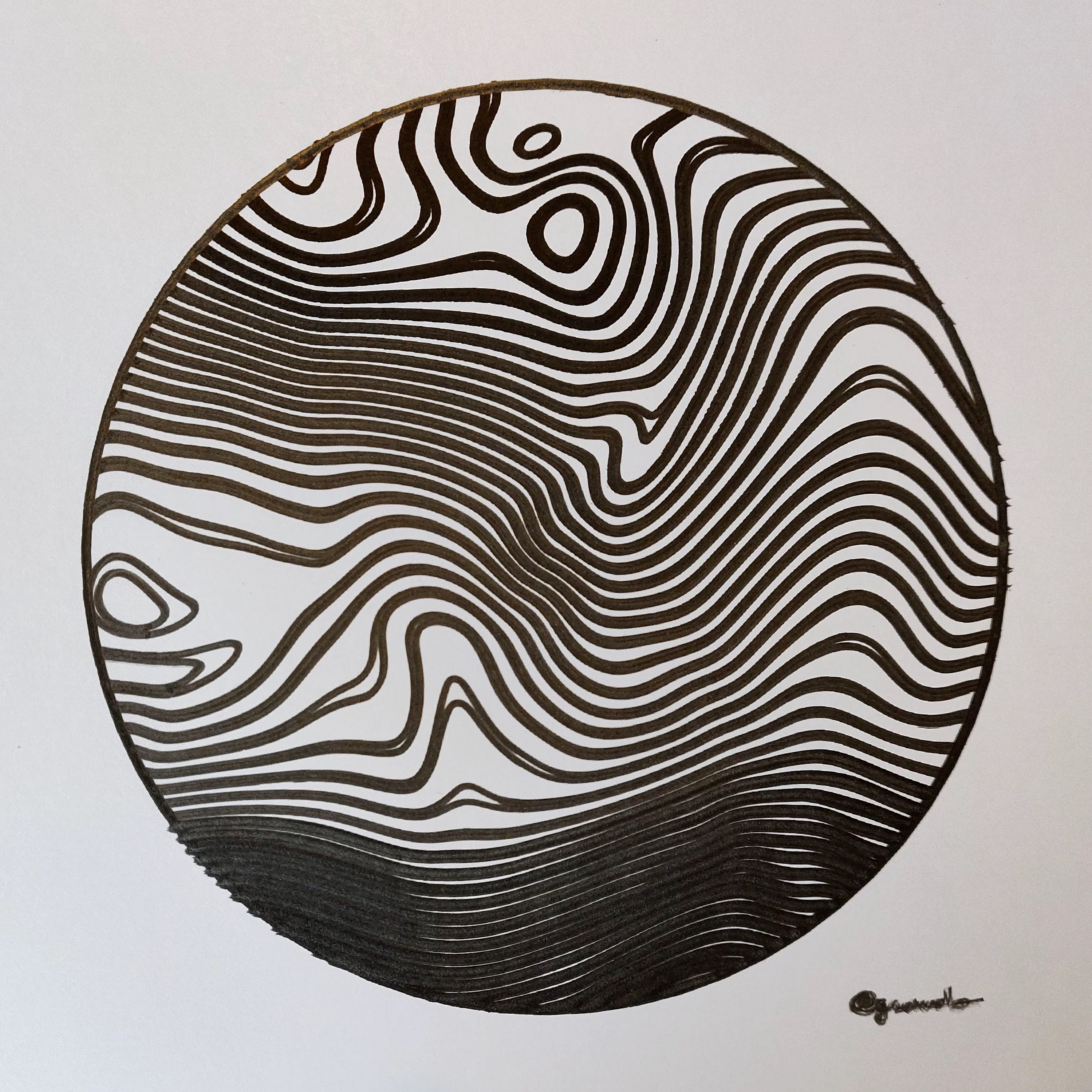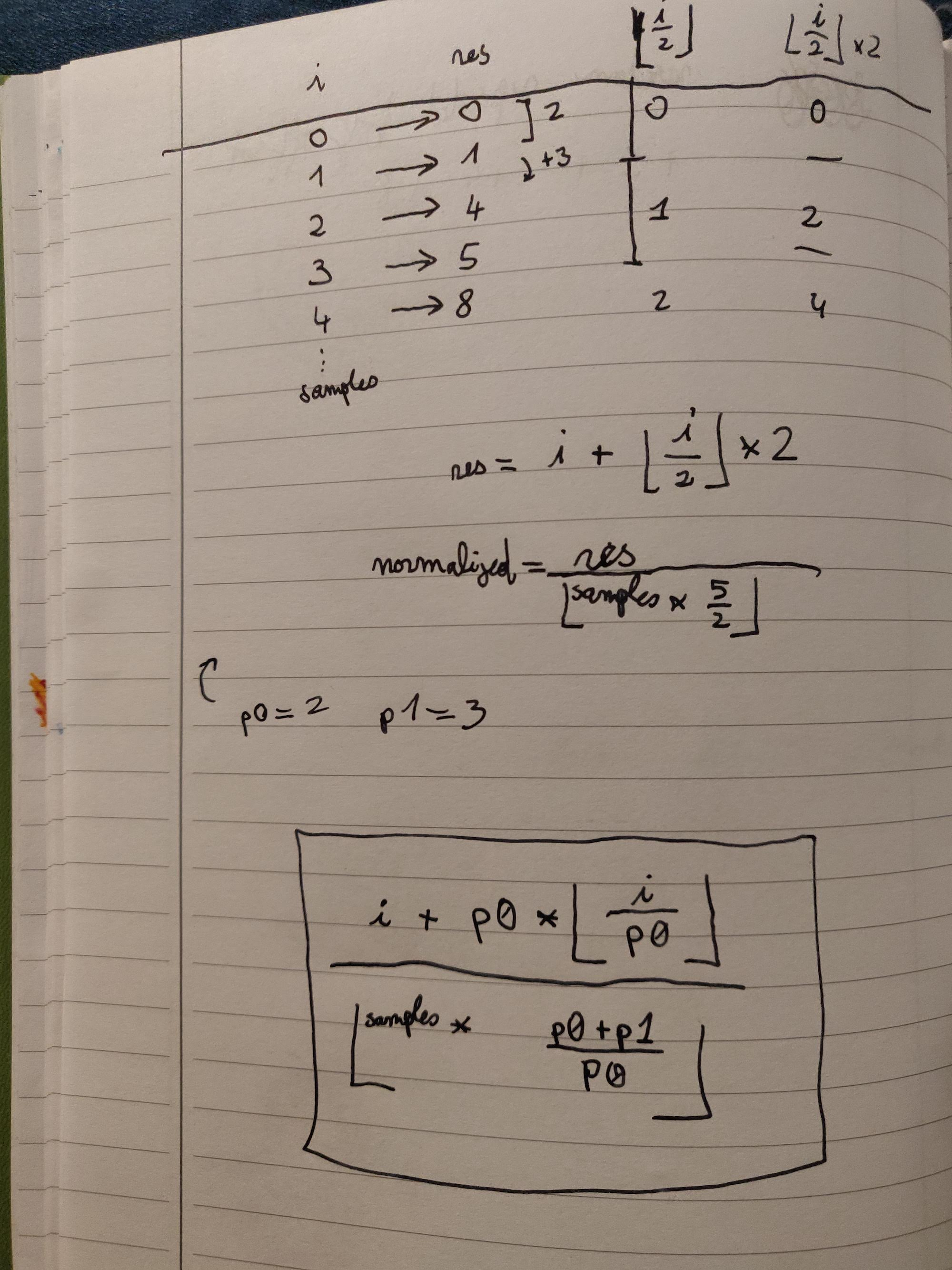# greweb.me / Plot #156 "Elevation 01"

Sourcecode
156/main.rsFirst experiment of Molotow pen on Bristol paper.

Using perlin noise and domain warping, we can make very interesting shapes that we can pipe into marching squares contour algorithm.

In this code, i've also made 2 lines close to each other instead of a linear distribution. This is relatively simple to implement:

``````let pattern = (2., 3.); // 2 lines 3 blanks
let thresholds: Vec<f64> = // in [0..1] range
(0..samples)
.map(|i|
(i as f64 + pattern.1 * (i as f64 / pattern.0).floor())
/ (samples as f64 * (pattern.0 + pattern.1) / pattern.0).floor())
.collect();
``````

Here is the formula: (that i'm not sure is correctly normalized by the way)

https://www.desmos.com/calculator/mlngfvssv0

(yeah I also use my fountain pen to do (simple) math =D)creative coder experimenting with GLSL shaders, Rust, and fountain pens robot plots. infinite noise explorer.